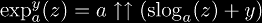# Identities

This is a complete rewrite of the previous identities page. All of the series expansions have been moved to the Special Functions pages.

# Notations

There are an unnecessarily large number of notations in common use for functions related to tetration. Ideally, only the most powerful notations would be used, however, as a matter of convinience we use left-superscript notation in preference to arrow notation and tower notation. Also, even though box notation is closer to hyper-operation terminology, arrow notation will be used, because it is more well-known.

• Arrow notation is used to write hyper-operations, and looks like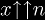for tetration. For more information, see the Hyperops page.
• Iteration notation is used to write iterated functions, and looks like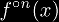• Quasigroup notation is used to write inverse functions of binary operations, and it looks like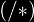(for hyper-roots) and(for hyper-logs).
• Section notation is used to write binary operations applied to only one argument, and it looks like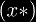or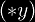. For more information, see Haskell sections.
• Tower notation is used to write nested exponentials, and looks like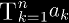• When all exponents are equal, and the top is 1, this can be written with left-superscript notation for tetration.
• When all exponents are equal except for the top, then this can be written with iterated exponential notation.

When choosing a notation, keep in mind your audience, and familiarity with tetration. In general, tetration should be written with left-superscript notation. If there is confusion with multiples in front, then use tower notation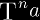, or if there is a complicated expression in the height, then use arrow notation.

# Axioms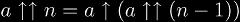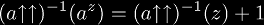# Infinite Tetrate and Company

The infinite tetrate is topologically conjugate to the 2nd super-root and the Lambert W-function.for more information see the section on topological conjugacy.

# Table of Tetration

For integer values: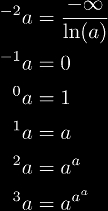For infinite values: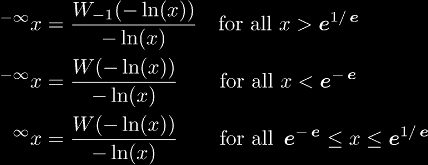# Table of Iterated Exponentials

For integer values from any x: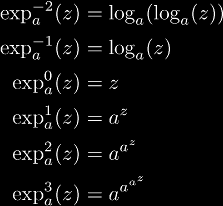For integer values from -1:# Inverse Functions

Notations for super-roots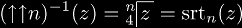Notations for super-logs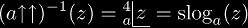# Miscellaneous

Exponentiation can be expressed in terms of exp and log: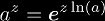Exponentiation can be expressed in terms of tet and slog: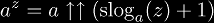Iterated exponentials can be expressed in terms of tet and slog: